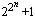# Fermat number

Pierre de Fermat wrote to Marin Mersenne on December 25, 1640 that:

If I can determine the basic reason why
3, 5, 17, 257, 65 537, ...,
are prime numbers, I feel that I would find very interesting results, for I have already found marvelous things [along these lines] which I will tell you about later. [Archibald1914].

This is usually taken to be the conjecture that every number of the formis prime.  So we call these the Fermat numbers, and when a number of this form is prime, we call it a Fermat prime.

The only known Fermat primes are the first five Fermat numbers: F0=3, F1=5, F2=17, F3=257, and F4=65537.  A simple heuristic shows that it is likely that these are the only Fermat primes (though many folks like Eisenstein thought otherwise).

In 1732 Euler discovered 641 divides F5. It only takes two trial divisions to find this factor because Euler showed that every divisor of a Fermat number Fn with n greater than 2 has the form k.2n+1+1 (exponent improved to n+2 by Lucas).  In the case of F5 this is 128k+1, so we would try 257 and 641 (129, 385, and 513 are not prime).  Now we know that all of the Fermat numbers are composite for the other n less than 31.  The quickest way to check a Fermat number for primality (if trial division fails to find a small factor) is to use Pepin's test.

Gauss proved that a regular polygon of n sides can be inscribed in a circle with Euclidean methods (e.g., by straightedge and compass) if and only if n is a power of two times a product of distinct Fermat primes.

The Fermat numbers are pairwise relatively prime, as can be seen in the following identity:

F0F1F2.....Fn-1 +2 = Fn.

(This gives a simple proof that there are infinitely many primes.)

The quickest way to find out if a Fermat number is prime, is to use Pepin's test [Pepin77]. It is not yet known if there are infinitely many Fermat primes, but it seems likely that there are not.

The book [KLS2001] is an excellent source of information on these numbers.

References:

Archibald1914
R. C. Archibald, "Remarks on Klien's `Famous problems of elementary geometry'," Amer. Math. Monthly, 21 (1914) 247--259.
Carmichael19
R. D. Carmichael, "Fermat numbers Fn = 22n + 1," Amer. Math. Monthly, 26 (1919) 137--146.
CDNY95
R. Crandall, J. Doenias, C. Norrie and J. Young, "The twenty-second Fermat number is composite," Math. Comp., 64 (1995) 863--868.  MR 95f:11104
CMP2003
R. E. Crandall, E. W. Mayer and J. S. Papadopoulos, "The twenty-fourth Fermat number is composite," Math. Comp., 72 (2003) 1555--1572. (Abstract available)
Gostin95
G. B. Gostin, "New factors of Fermat numbers," Math. Comp., 64 (1995) 393--395.  MR 95c:11151
HB1975
J. C. Hallyburton, Jr. and J. Brillhart, "Two new factors of Fermat numbers," Math. Comp., 29 (1975) 109--112.  Collection of articles dedicated to Derrick Henry Lehmer on the occasion of his seventieth birthday.  MR 51:5460
HS61
A. Hurwitz and J. L. Selfridge, "Fermat numbers and perfect numbers," Notices Amer. Math. Soc., 8 (1961) 601.  Abstract 587-104.
Keller83
W. Keller, "Factors of Fermat numbers and large primes of the form k· 2n +1," Math. Comp., 41 (1983) 661-673.  MR 85b:11117
Keller92
W. Keller, "Factors of Fermat numbers and large primes of the form k· 2n + 1 ii," Hamburg, (September 1992) Manuscript.
KLS2001
M. Krízek, F. Luca and L. Somer, 17 lectures on Fermat numbers: from number theory to geometry, CMS Books in Mathematics Vol, 9, Springer-Verlag, New York, NY, 2001.  pp. xvii + 257, ISBN 0-387-95332-9. MR 2002i:11001
LLMP93
A. K. Lenstra, Lenstra, Jr., H. W., M. S. Manasse and J. M. Pollard, "The factorization of the ninth Fermat number," Math. Comp., 61 (1993) 319-349.  Addendum, Math. Comp. 64 (1995), 1357.  MR 1 303 085
Pepin77
T. Pepin, "Sur la formule 22n+1," C. R. Acad. Sci. Paris, 85 (1877) 329-331.
Robinson54
R. M. Robinson, "Mersenne and Fermat numbers," Proc. Amer. Math. Soc., 5 (1954) 842-846. [Announces the discovery of the 13th through 17th Mersenne primes--the first Mersenne primes found by electronic computer.]
Robinson57
R. M. Robinson, "Factors of Fermat numbers," Math. Tables Aids Comput., 11 (1957) 21-22.
Robinson58
R. M. Robinson, "A report on primes of the form k· 2n + 1 and on factors of Fermat numbers," Proc. Amer. Math. Soc., 9 (1958) 673--681.  MR 20:3097
Selfridge53
J. L. Selfridge, "Factors of Fermat numbers," Math. Tables Aids Comput., 7 (1953) 274-275.
Shippee1978
D. E. Shippee, "Four new factors of Fermat numbers," Math. Comp., 32:143 (1978) 941. (Abstract available)
SS1981
Shorey, T. N. and Stewart, C. L., "On divisors of Fermat, Fibonacci, Lucas and Lehmer numbers. II," J. London Math. Soc. (2), 23:1 (1981) 17--23.  MR 82m:10025
Stewart1977
C. L. Stewart, "On divisors of Fermat, Fibonacci, Lucas and Lehmer numbers," Proc. Lond. Math. Soc., 35:3 (1977) 425--447.  MR 58:10694
Stewart1983
Stewart, C. L., "On divisors of Fermat, Fibonacci, Lucas and Lehmer numbers. III," J. London Math. Soc. (2), 28:2 (1983) 211--217.  MR 85g:11021
Stewart1983
Stewart, C. L., "On divisors of Fermat, Fibonacci, Lucas and Lehmer numbers. III," J. London Math. Soc. (2), 28:2 (1983) 211--217.  MR 85g:11021
Wrathall64
C. P. Wrathall, "New factors of Fermat numbers," Math. Comp., 18 (1964) 324--325.  MR 29:1167
YB88
J. Young and D. A. Buell, "The twentieth Fermat number is composite," Math. Comp., 50 (1988) 261--263.  MR 89b:11012
Young98
J. Young, "Large primes and Fermat factors," Math. Comp., 67:244 (1998) 1735--1738.  MR 99a:11010
Abstract: A systematic search for large primes has yielded the largest Fermat factors known.